Case Based Questions Test: Quadratic Equations

# Case Based Questions Test: Quadratic Equations

Test Description

## 15 Questions MCQ Test Mathematics (Maths) Class 10 | Case Based Questions Test: Quadratic Equations

Case Based Questions Test: Quadratic Equations for Class 10 2022 is part of Mathematics (Maths) Class 10 preparation. The Case Based Questions Test: Quadratic Equations questions and answers have been prepared according to the Class 10 exam syllabus.The Case Based Questions Test: Quadratic Equations MCQs are made for Class 10 2022 Exam. Find important definitions, questions, notes, meanings, examples, exercises, MCQs and online tests for Case Based Questions Test: Quadratic Equations below.
Solutions of Case Based Questions Test: Quadratic Equations questions in English are available as part of our Mathematics (Maths) Class 10 for Class 10 & Case Based Questions Test: Quadratic Equations solutions in Hindi for Mathematics (Maths) Class 10 course. Download more important topics, notes, lectures and mock test series for Class 10 Exam by signing up for free. Attempt Case Based Questions Test: Quadratic Equations | 15 questions in 30 minutes | Mock test for Class 10 preparation | Free important questions MCQ to study Mathematics (Maths) Class 10 for Class 10 Exam | Download free PDF with solutions
 1 Crore+ students have signed up on EduRev. Have you?
Case Based Questions Test: Quadratic Equations - Question 1

### Read the following text and answer the following questions on the basis of the same: Nidhi and Ria are very close friends. Nidhi’s parents own a Maruti Alto and Ria’s parents own a Toyota Liva. Both the families decided to go for picnic to Somnath temple in Gujarat by their own cars. Nidhi car travels x km/h when Ria’s car travels 5 km/h more than Nidhi’s car Nidhi’s car took 4 hours more than Ria’s car in covering 400 km.Q. Which of the following quadratic equations describe the speed of Nidhi’s car?

Detailed Solution for Case Based Questions Test: Quadratic Equations - Question 1

Let Speed of Nidhi = x km/h

Time taken = t + 4 km/h

Time = D/s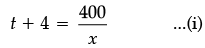Speed of Ria = (x + 5) km/h

Time taken = t hour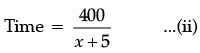According to the question,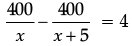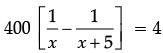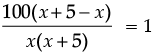⇒ x2 + 5x – 500 = 0

Case Based Questions Test: Quadratic Equations - Question 2

### Read the following text and answer the following questions on the basis of the same:Nidhi and Ria are very close friends. Nidhi’s parents own a Maruti Alto and Ria’s parents own a Toyota Liva. Both the families decided to go for picnic to Somnath temple in Gujarat by their own cars. Nidhi car travels x km/h when Ria’s car travels 5 km/h more than Nidhi’s car Nidhi’s car took 4 hours more than Ria’s car in covering 400 km.Q. How much time took Ria to travel 400 km?

Detailed Solution for Case Based Questions Test: Quadratic Equations - Question 2 Ria’s speed = 20 + 5 = 25 km/h

Distance = 400 km

Time = D/S = 400/25 = 16 hour

Case Based Questions Test: Quadratic Equations - Question 3

### Read the following text and answer the following questions on the basis of the same:Nidhi and Ria are very close friends. Nidhi’s parents own a Maruti Alto and Ria’s parents own a Toyota Liva. Both the families decided to go for picnic to Somnath temple in Gujarat by their own cars. Nidhi car travels x km/h when Ria’s car travels 5 km/h more than Nidhi’s car Nidhi’s car took 4 hours more than Ria’s car in covering 400 km.Q. What will be the distance covered by Ria’s car in two hours?

Detailed Solution for Case Based Questions Test: Quadratic Equations - Question 3 We know that distance = Speed × time

= (x + 5) × 2 = 2(x + 5) km

Case Based Questions Test: Quadratic Equations - Question 4

Read the following text and answer the following questions on the basis of the same:

Nidhi and Ria are very close friends. Nidhi’s parents own a Maruti Alto and Ria’s parents own a Toyota Liva. Both the families decided to go for picnic to Somnath temple in Gujarat by their own cars. Nidhi car travels x km/h when Ria’s car travels 5 km/h more than Nidhi’s car Nidhi’s car took 4 hours more than Ria’s car in covering 400 km.Q. What is the speed of Nidhi’s car?

Detailed Solution for Case Based Questions Test: Quadratic Equations - Question 4 x2 + 5x – 500 = 0

⇒ x2 + 25x – 20x – 500 = 0

⇒ x (x + 25) – 20 (x + 25) = 0

⇒ (x + 25)(x – 20) = 0 ⇒ x = – 25 (Rejected) and 20

Case Based Questions Test: Quadratic Equations - Question 5

Read the following text and answer the following questions on the basis of the same:

Nidhi and Ria are very close friends. Nidhi’s parents own a Maruti Alto and Ria’s parents own a Toyota Liva. Both the families decided to go for picnic to Somnath temple in Gujarat by their own cars. Nidhi car travels x km/h when Ria’s car travels 5 km/h more than Nidhi’s car Nidhi’s car took 4 hours more than Ria’s car in covering 400 km.Q. Name the shape of quadratic equation in graph
Detailed Solution for Case Based Questions Test: Quadratic Equations - Question 5 The graph of a quadratic function is called a parabola and has a curved shape. One of the main points of a parabola is its vertex. It is the highest or the lowest point on its graph. You can think of like an endpoint of a parabola.
Case Based Questions Test: Quadratic Equations - Question 6

Read the following text and answer the following questions on the basis of the same: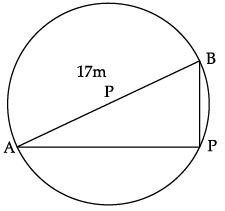Aniket is studying in X standard. He is created a pole at on the boundary of a circular park of diameter 17 m in such a way that the difference of its distances from two diametrically opposite fixed gates A and B on the boundary is 7m.

Q. Find the distance between pole and gate B.
Detailed Solution for Case Based Questions Test: Quadratic Equations - Question 6 x2 + 7x – 120 = 0

x2 + 15x – 8x – 120 = 0

x (x + 15) – 8 (x + 15) = 0

x = 8 and x = – 15 (rejected)

Case Based Questions Test: Quadratic Equations - Question 7

Read the following text and answer the following questions on the basis of the same:Aniket is studying in X standard. He is created a pole at on the boundary of a circular park of diameter 17 m in such a way that the difference of its distances from two diametrically opposite fixed gates A and B on the boundary is 7m.

Q. What is the length of (AP + BP)?

Detailed Solution for Case Based Questions Test: Quadratic Equations - Question 7 AP + BP = 15 + 8 = 23 cm
Case Based Questions Test: Quadratic Equations - Question 8

Read the following text and answer the following questions on the basis of the same:Aniket is studying in X standard. He is created a pole at on the boundary of a circular park of diameter 17 m in such a way that the difference of its distances from two diametrically opposite fixed gates A and B on the boundary is 7m.

Q. Find a quadratic equations in variable x for above situation.
Detailed Solution for Case Based Questions Test: Quadratic Equations - Question 8 Let the distance of the pole from the gate B be x i.e. BP = x cm.

Therefore, AP = x + 7 m Now, AB = 17 m and since AB is a diameter.

Apply Pythagoras theorem,

AB2 = AP2 + BP2

172 = (x + 7)2 + x2

289 = x2 + 49 + 14x + x2

x2 + 7x – 120 = 0

Case Based Questions Test: Quadratic Equations - Question 9

Read the following text and answer the following questions on the basis of the same:Aniket is studying in X standard. He is created a pole at on the boundary of a circular park of diameter 17 m in such a way that the difference of its distances from two diametrically opposite fixed gates A and B on the boundary is 7m.

Q. Find the distance between pole and gate A.
Detailed Solution for Case Based Questions Test: Quadratic Equations - Question 9 AP = x + 7 = 8 + 7 = 15 cm
Case Based Questions Test: Quadratic Equations - Question 10

Read the following text and answer the following questions on the basis of the same:Aniket is studying in X standard. He is created a pole at on the boundary of a circular park of diameter 17 m in such a way that the difference of its distances from two diametrically opposite fixed gates A and B on the boundary is 7m.

Q. Find the area of triangle ABP.
Detailed Solution for Case Based Questions Test: Quadratic Equations - Question 10 Area of triangle ABP = 1/2 × 23 × 8 = 92 cm2.
Case Based Questions Test: Quadratic Equations - Question 11

Read the following text and answer the following questions on the basis of the same:

A small scale industry produces a certain boxes of candles in a day. Number of boxes prepared by each worked on a particular day was 2 more than thrice the number of workers working in the industry. The number of boxes produced in a particular day was 85.

Q. Represent the above equation in quadratic equation.

Detailed Solution for Case Based Questions Test: Quadratic Equations - Question 11 The Correct equation Formed

3x2 + 2x – 85

Case Based Questions Test: Quadratic Equations - Question 12

Read the following text and answer the following questions on the basis of the same:

A small scale industry produces a certain boxes of candles in a day. Number of boxes prepared by each worked on a particular day was 2 more than thrice the number of workers working in the industry. The number of boxes produced in a particular day was 85.

Q. Number of workers working in the industry:

Detailed Solution for Case Based Questions Test: Quadratic Equations - Question 12 Let the workers are x, Number of boxes prepared by the workers

= 2 + 3x

According to the question,

x(2 + 3x) = 85

⇒ 3x2 + 2x – 85 = 0

⇒ 3x2 + 17x – 15x – 85 = 0

⇒ x(3x + 17) – 5(3x + 17) = 0 ⇒

⇒ (3x + 17)(x – 5) = 0

⇒ x = 5

Case Based Questions Test: Quadratic Equations - Question 13

Read the following text and answer the following questions on the basis of the same:

A small scale industry produces a certain boxes of candles in a day. Number of boxes prepared by each worked on a particular day was 2 more than thrice the number of workers working in the industry. The number of boxes produced in a particular day was 85.

Q. If the number of workers working in the industry is x. What was the number of boxes of candles prepared by each worker on that particular day?

Detailed Solution for Case Based Questions Test: Quadratic Equations - Question 13 Let the workers are x, Number of boxes prepared by the workers

= 2 + 3x

According to the question,

x(2 + 3x) = 85

⇒ 3x2 + 2x – 85 = 0

⇒ 3x2 + 17x – 15x – 85 = 0

⇒ x(3x + 17) – 5(3x + 17) = 0 ⇒

⇒ (3x + 17)(x – 5) = 0

⇒ x = 5

Case Based Questions Test: Quadratic Equations - Question 14

Read the following text and answer the following questions on the basis of the same:

A small scale industry produces a certain boxes of candles in a day. Number of boxes prepared by each worked on a particular day was 2 more than thrice the number of workers working in the industry. The number of boxes produced in a particular day was 85.

Q. Nature of roots of the above quadratic equation are:

Detailed Solution for Case Based Questions Test: Quadratic Equations - Question 14 We can see, the discriminant of the given quadratic equation is positive but not a perfect square. Hence, the roots of a quadratic equation are real, unequal.
Case Based Questions Test: Quadratic Equations - Question 15

Read the following text and answer the following questions on the basis of the same:

A small scale industry produces a certain boxes of candles in a day. Number of boxes prepared by each worked on a particular day was 2 more than thrice the number of workers working in the industry. The number of boxes produced in a particular day was 85.

Q. How many boxes will be prepared when number of workers are increased by 2.

Detailed Solution for Case Based Questions Test: Quadratic Equations - Question 15 Number of workers = x

Number of workers when increased by 2 = x + 2

∴ Number of boxes prepared by the workers

(x + 2) = (x + 3) [2 + 3 (x + 2)

= 7 [2 + 3 × 7] [∵ x = 5]

= 7 × 23

= 161.

## Mathematics (Maths) Class 10

53 videos|369 docs|138 tests
 Use Code STAYHOME200 and get INR 200 additional OFF Use Coupon Code
Information about Case Based Questions Test: Quadratic Equations Page
In this test you can find the Exam questions for Case Based Questions Test: Quadratic Equations solved & explained in the simplest way possible. Besides giving Questions and answers for Case Based Questions Test: Quadratic Equations, EduRev gives you an ample number of Online tests for practice

## Mathematics (Maths) Class 10

53 videos|369 docs|138 tests